PROVIDING ACCURATE, RELIABLE AND STRAIGHT TO THE POINT KNOWLEDGE ABOUT DIFFERENT IT TOOLS EXPLAINED WITH PRACTICAL EXAMPLES

# MANIPULATING TEXT IN EXCEL PART-II

## INTRODUCTION

Whenever we prepare any report in excel, we have two constituents in any report.

The Text portion and the Numerical portion.

But just storing the text and numbers doesn’t make the super reports. Many times we need to automate the process in the reports to minimize the effort and improve the accuracy.

Many functions are provided by the Excel which work on Text and give us the useful output as well. But few problems are still left on which we need to apply some tricks with the available tools.

THIS WAS AN EXCERPT FROM THE FIRST ARTICLE OF THIS SERIES MANIPULATING TEXT IN EXCEL – PART I

In this articles , we will continue learning many more techniques about the manipulation of text in Excel.

## HOW TEXT IS HANDLED IN EXCEL?

TEXT is simply the group of characters and strings of characters which convey the information about the different data and numbers in Excel. Every character is connected with a code [ANSI].
Text comprises of the individual entity character which is the smallest bit which would be found in Excel.
We can perform the operations on the strings[Text] or the characters.
Characters are not limited to A to Z or a to z but many symbols are also included in this which we would see in the later part of the article.

TEXT IS AN INACTIVE NUMBER TYPE[FORMAT] IN EXCEL. ANYTHING STORED AS TEXT [NUMBER OR DATE] WON’T RESPOND TO ANY STANDARD FORMULAS OR FUNCTIONS BUT SPECIALLY DESIGNED TEXT FUNCTIONS. [EXCEPTIONS DO OCCUR IN CASE OF NUMBERS]
If we need to make anything inactive, such as Date to be non responding to the calculation, we put it as a text. Similarly if we want to avoid any calculations for a number it needs to be put as a text.

## CONDITIONAL FORMATTING BASED ON TEXT

CONDITIONAL FORMATTING is the process of formatting in Excel on the basis of the conditions. We can put many conditions in the cell and program the Excel to make the formatting , as desired, if the particular condition is met. Formatting comprises of the foreground color, background color, font, size etc. which are the properties of the text.

It makes the results more readable.

IT IS RECOMMENDED TO LEARN THE BASICS ABOUT CONDITIONAL FORMATTING, IF NOT VERY COMFORTABLE WITH THIS TOPIC.

Maximum times, we apply the conditional formatting on the basis of values present in the cells. Let us now learn the way by which we can apply the conditional formatting using the Text.

HIGHLIGHTING THE TEXT EQUAL TO SOME VALUE:

Let us find out the cell which contains a text value equal to some SPECIFIED TEXT.

For the example let us take this block of text values in Excel.

 HELLO HOW ARE YOU RED GREEN YELLOW BLUE GREY WHITE BIG BUFFALO ZEBRA XYLOPHONE SMALL BLACK APPLE MANGO GUAVA ONION PUMPKIN OKRA KIWI POTATO SPRING

Let us try to highlight the cells containing HELLO.

It can be done in two ways.

1. Using the predefined options.

2. Using custom formula.

STEPS to highlight cells using the predefined option:

•  Select the complete table.
• Go to HOME TAB>CONDITIONAL FORMATTING>HIGHLIGHT CELL RULES>EQUAL TO
• Click EQUAL TO.
• Following dialog box will open.
• Enter the value in the field as shown in the picture.
• Select the format of the cells which satisfy the condition.
• and Click OK.
• After clicking OK, we will see the result as the cell with HELLO will be in pink. [The option doesn’t differ the cases. Both of upper and lower cases will be taken as same.]
• Similarly we can find other values too.

2. Using custom formula.

STEPS to highlight cells using the custom formula:

•  Select the complete table.
• Go to HOME TAB>CONDITIONAL FORMATTING>NEW RULE.
• A dialog box as shown in the picture below will open.
• Click the last option: use a formula to determine which cells to format.
•  Enter the formula in the field as =E6=”HELLO”.
• Set the format as per your choice. We set the fill color as green.[Don’t forget to change the format otherwise difference won’t be visible.]
• Click OK.
• The result will be highlighted as shown in the picture below.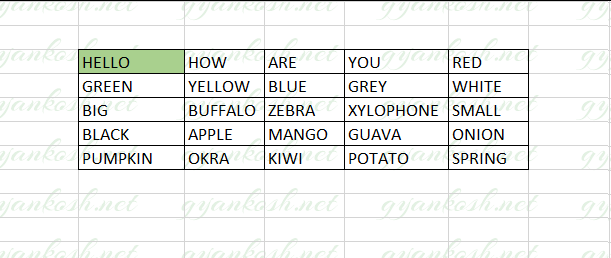### EXAMPLE 2:STEPS TO  HIGHLIGHT THE TEXT MATCHING THE GIVEN TEXT [EXACT MATCH]

In this example, let us find out the way to highlight the cells which contains the text same as some specified text exactly with the same content and with the same case.

GENERALIZED FORMULA TO HIGHLIGHT THE EXACT TEXT:
=EXACT(FIRST CELL OF SELECTION, “TEXT TO BE MATCHED”).
For our example, we will highlight the cell containing the text “Yellow”.
• Select the complete table.
• Go to HOME TAB>CONDITIONAL FORMATTING>NEW RULE.
• A dialog box as shown in the picture below will open.
• Click the last option: use a formula to determine which cells to format.
• Enter the formula =EXACT(E6,”Yellow”).
• Choose the format of your choice, which will appear when some cell fulfill the condition.
• Click OK.

### EXAMPLE 3: STEPS TO  HIGHLIGHT THE CELLS IF TEXT CONTAIN A SPECIFIC CHARACTER

Suppose we want to create a condition when we want to highlight the cell if the cell contains any specific character say # for our example.
GENERALIZED FORMULA TO HIGHLIGHT THE CELLS WHICH CONTAIN A SPECIFIC CHARACTER
• Select the complete table.
• Go to HOME TAB>CONDITIONAL FORMATTING>NEW RULE.
• A dialog box as shown in the picture below will open.
• Click the last option: use a formula to determine which cells to format.

## TRUNCATE TEXT IN EXCEL

TRUNCATING TEXT in Excel means to cut it down from the various places as per our requirement.

We can take it further creating the task where we can remove any text portion after a certain character such as comma or space or full stop.

So, in Excel we have got some cutting tools which we already discussed in our previous post. [MANIPULATING TEXT IN MICROSOFT EXCEL PART I]

We can do so using the standard and powerful text truncating tools RIGHT, LEFT and MID functions easily.

There are complete articles on each of them how to use and other information. Visit them by clicking the FUNCTION NAME given here.

LEFT FUNCTION has the capability to pick the specified number of characters from the left side of the text.

RIGHT FUNCTION can extract the specified number of characters from the right whereas

MID FUNCTION takes out the specified number of the characters from the middle of the text.

We consider that you are familiar with the functions now.

Let us discuss a few examples how we can extract text.

LET US TAKE A TEXT FOR THE EXAMPLE.

“WOW! IT IS A WONDERFUL DAY” in cell E7.

EXAMPLE 1: TRUNCATE THE TEXT AFTER 5 LETTERS FROM THE LEFT.

GENERALIZED FORMULA TO BE USED =LEFT(CELL CONTAINING TEXT, NUMBER OF CHARACTERS TO BE KEPT FROM THE LEFT)

### STEPS TO TRUNCATE TEXT AFTER 5 LETTERS FROM THE LEFT.

• Put the text in a cell.
• Select the cell where we want the result.
•  Enter the formula =LEFT(E7,5)
• The result would appear as WOW! . [THERE IS A SPACE AFTER ‘!’]
• Look at the picture below for the description.
WHEN IT COMES TO CHARACTERS, SPACE ITSELF IS A CHARACTER. ALWAYS TAKE ITS CARE AS NEGLECTING THE SPACE CAUSES MUCH PROBLEMS SOMETIMES.

THE EXAMPLE.

“WOW! IT IS A WONDERFUL DAY” in cell E7.

### EXAMPLE 2: TRUNCATE THE TEXT FROM THE LEFT AND REMOVE FIRST 5 LETTERS.

GENERALIZED FORMULA TO BE USED TO TRUNCATE THE TEXT FROM THE LEFT FOR A FIXED NUMBER OF CHARACTERS IS

=RIGHT(CELL CONTAINING TEXT, LEN(CELL CONTAINING TEXT) -NUMBER OF CHARACTERS TO BE KEPT FROM THE LEFT)

For the example, we’ll remove the first 5 characters from the left.

### STEPS TO TRUNCATE TEXT FOR FIVE CHARACTERS FROM THE LEFT.

• Put the text in a cell.
• Select the cell where we want the result.
•  Enter the formula =RIGHT(E7,LEN(E7)-5)
• The result would appear as WHAT A WONDERFUL DAY . [ THE REMOVAL HAS BEEN DONE FOR THE FIRST FIVE CHARACTERS].
• Look at the picture below for the description.

THE EXAMPLE.

“WOW! IT IS A. WONDERFUL DAY” in cell E18.

### EXAMPLE 3: TRUNCATE THE TEXT AFTER A CERTAIN CHARACTER LIKE COMMA OR FULL STOP.

GENERALIZED FORMULA TO BE USED TO TRUNCATE THE TEXT FROM THE LEFT FOR A FIXED NUMBER OF CHARACTERS IS

=LEFT(CELL CONTAINING TEXT, SEARCH(” CHARACTER” , CELL CONTAINING TEXT, 1)

For the example, we’ll remove the text after the full stop.

### STEPS TO TRUNCATE TEXT AFTER FULL STOP.

• Put the text in a cell.
• Select the cell where we want the result.
•  Enter the formula =LEFT(E18,SEARCH(“.”,E18,1))
• The result would appear as WOW! IT IS A. [ THE REMOVAL HAS BEEN DONE FOR THE TEXT AFTER THE FULL STOP].
• Look at the picture below for the description and result.
This was an example for the ‘.’ full stop. But we can apply it to any type of special character or even spaces.

## CREATE VERTICAL TEXT IN EXCEL

Let us try to create vertical text in excel.

The vertical text can be the need of any report where we want the heading to be vertical.

TEXT BEING VERTICAL can have two meanings.

1. Vertical text but in the same cell.

2. Vertical text in the different cells.

VERTICAL TEXT IN THE SAME CELL:

Follow the steps to create a vertical text in the same cell.

1. Enter the text normally, where we want to enter.

2. Go to HOME TAB>ORIENTATION>VERTICAL TEXT.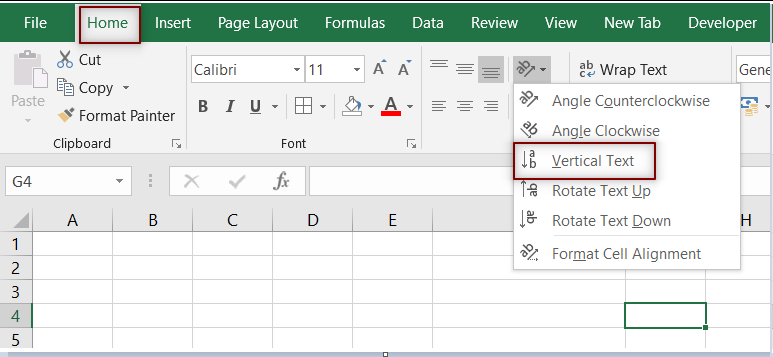3. The text will become vertical simply. Check out the following picture for the results.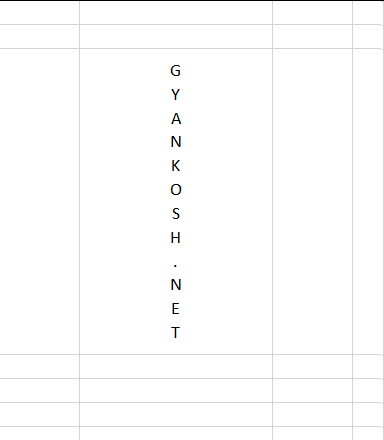2.VERTICAL TEXT IN DIFFERENT CELLS:

If the text is in cells horizontally and we want to place it vertically we can use the following methods.

Let us take an example where the following text is put in the cells horizontally.

HELLO as shown in the picture below.

USING PASTE SPECIAL

USING TRANSPOSE FUNCTION.

USING PASTE SPECIAL: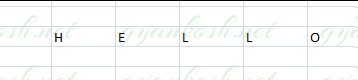STEPS TO MAKE THE TEXT VERTICAL USING THE PASTE SPECIAL FUNCTION

• Select all the cells containing the letters or words.
• Right Click the cell where we want to put the text vertically.
• Choose transpose option directly or paste special.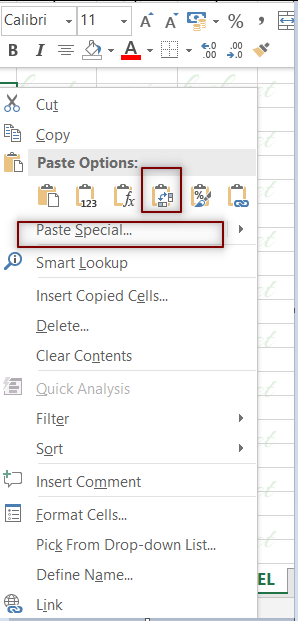• The result will appear.
• Instead if we go for PASTE SPECIAL. The paste special dialog box will open.
• Choose TRANSPOSE and click OK.

Click OK.

The result will appear as the word HELLO will become vertical .

### USING TRANSPOSE FUNCTION.

GIVEN TEXT- HELLO as shown in the picture below.STEPS TO MAKE THE TEXT VERTICAL USING THE TRANSPOSE FUNCTION.

• Select the exact number of cells in the same layout of the actual data which needs to be transposed i.e. with the same number of rows to be converted to same number of columns at a new place. Look at the picture above. We selected the exact 5 vertical cells before applying the function.(D18 TO H18 in this case)
• Now type the formula =TRANSPOSE(D18:H18) but don’t PRESS ENTER.
• Press CTRL+SHIFT+ENTER.
• The text will become vertical.

## CROSS OUT /STRIKETHROUGH TEXT IN EXCEL

This section deals with the techniques to STRIKETHROUGH or CROSS OUT the text in Excel.

STEPS TO STRIKETHROUGH OR CROSSOUT THE TEXT IN EXCEL.

Right Click the cell containing the text and choose FORMAT CELLS.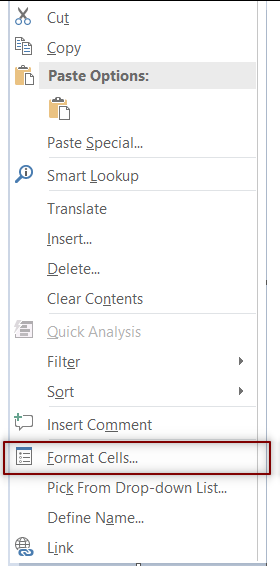In the dialog box opened, go to FONT TAB and click STRIKETHROUGH as shown in the picture below.

Click OK.

The text will be crossed out.

Look at the final position.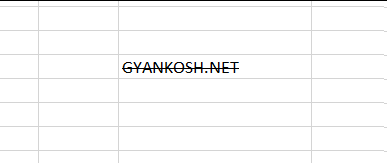SHORTCUT TO CROSS OUT TEXT / STRIKETHROUGH

We can simply use a simple shortcut to strikethrough or cross out the text by selecting the cell containing the text and pressing CTRL+5.

## APPEND TEXT IN EXCEL

Appending or attaching the text at the end of text is a frequent requirement in Excel. Also mixing our result with the text snippets and values is required.

Let us find out, how we can append or attach new text at the end.

It can be done in two major ways.

1. USING & OPERATOR.

2. USING CONCATENATE OR CONCAT FUNCTION.

USING & OPERATOR:

The ‘&’ operator is also known as concatenate operator. [CLICK HERE FOR MORE DETAILS ABOUT & CONCATENATE OPERATOR].

EXAMPLE:

Append “.net” after all the text given names.

The names given are

Dave

Abraham

John

Keval

STEPS TO APPEND TEXT IN EXCEL USING & OPERATOR

Select the cell where the appended text is needed.

Put the formula as =cell containing text&”text to be attended”

For our example , the formula will be =C6&”.net”

Drag down the formula through the column.

The result is shown in the picture below.

2. USING CONCATENATE OR CONCAT FUNCTION.

EXAMPLE:

Append “.com” after all the text given names.

The names given are

Dave

Abraham

John

Keval

STEPS TO APPEND TEXT IN EXCEL USING CONCAT AND CONCATENATE FUNCTION

• Select the cell where the appended text is needed.
• Put the formula as =CONCAT (cell containing text,”text to be attended”,”text2 to be appended ” and so on). Similarly we can also use =CONCATENATE (cell containing text,”text to be attended”,”text2 to be appended ” and so on)
• For our example , the formula will be =CONCAT(C6&,”.com”) and in few cases =CONCATENATE(C6,”.com”)
• Drag down the formula through the column.
• The result is shown in the picture below.

## COUNT UNIQUE TEXT VALUES IN EXCEL

This can be a requirement sometime when we need to count the unique text values in a given data or column. It can be done with a number of ways. Let us discuss a few.

1. USING REMOVE DUPLICATES

2. USING UNIQUE FUNCTION

USING REMOVE DUPLICATES:

This is an option already available in Excel directly and very easy to use.

THIS OPTION IS NOT AUTOMATIC AND SHOULD BE USED ONLY IF WE DON’T HAVE MUCH DATA TO BE ANALYZED.

STEPS TO USE REMOVE DUPLICATES TO COUNT THE UNIQUE TEXT VALUES IN EXCEL

[*KINDLY VISIT HERE FOR COMPLETE INFORMATION ABOUT THE PROCEDURE OF REMOVING DUPLICATES IN EXCEL]

Select the complete data or column where we want to find the number of unique values. [ If we need the original column intact, copy the column and paste it somewhere else. We can perform operations on this column].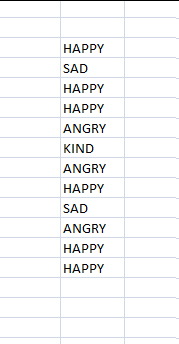Go to HOME>DATA>REMOVE DUPLICATES.

Select the column from the dialog box. We have only one column so choose the column.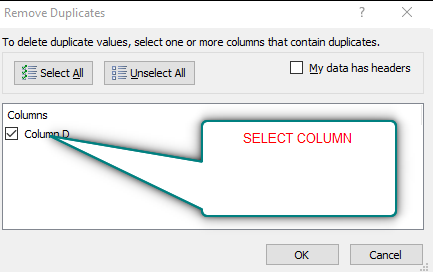After selecting the column, press OK. It’ll leave only unique values in the column.

Now , we can put the formula =COUNTA(FIRST CELL OF COLUMN: LAST CELL OF COLUMN).

It’ll give us the number of unique text values present in the column.

2. USING UNIQUE FUNCTION
In this method we will make use of UNIQUE FUNCTION which will help us to find out the unique values or texts in the given column. After getting all the unique values we can count them easily. Let us start.
We’ll take an example for trying our procedure.
Suppose we have the following data.STEPS TO COUNT UNIQUE TEXT VALUES IN A EXCEL USING UNIQUE FUNCTION.

[THE COMPLETE PICTORIAL REPRESENTATION OF THE USE OF UNIQUE FUNCTION IS HERE].

We have to process the information twice to get the desired results. First of all we need to find the unique values.

• Select the cell where we want to find the unique values .
• Put the following formula.
• =UNIQUE(ARRAY,COLUMN WISE, FALSE)
• For our example the formula will be
• =UNIQUE(G6:G17,FALSE,0) [The first argument is the array containing the data. The second argument tell it that it has to do the comparison in the rows and third tells that it can count the entries which are more than one].
• The result array will contain only the unique values.
• In the next column we can count these by the use of COUNTA FUNCTION as =COUNTA(H6:H17). We can see in the picture that we had only 4 values but we took a wide range. The reason is that we don’t know what is the number of unique values if the data is too large.
• Take a look at the picture below for the reference.

## EXTRACT SPECIFIC PORTION OF A TEXT IN EXCEL

If we need to extract some specific portion of a text in Excel, we can use our well known MID FUNCTION for this easily.

STEPS TO EXTRACT SPECIFIC PORTION OF A TEXT IN EXCEL

For this option, we can have two situations.

1. We know the starting and ending character number of the portion wanted.

2. We don’t know the character number of the portion to be extracted but we know the condition.

STEPS TO EXTRACT TEXT IF WE KNOW THE CHARACTER POSITION:

We can simply use the MID FUNCTION for this situation.

EXAMPLE:

Extract the text from character 5 to 7 from the given text.

how are you?

Suppose we have the given text in cell E7.

Select the cell where we want the output.

Put the formula as =MID(E7,5,7)

The result would appear as ‘are’.

The process is shown in the picture below.

## COUNTIF WITH TEXT VALUES IN EXCEL

COUNTIF FUNCTION counts the values with certain given conditions for example, if any number is smaller than a particular value, or larger than a fixed value or equal to any text etc.

In this section we would focus upon the text portion.

Let us find out with the help of examples, how we can apply COUNTIF to extract useful information from the data.

EXAMPLE 1: COUNTING THE NUMBER OF REPETITIONS OF A PARTICULAR TEXT IN EXCEL

WASHINGTON

PARIS

DELHI

DELHI

WASHINGTON

MOSCOW

ROME

ROME

MOSCOW

STEPS TO FIND THE NUMBER OF REPETITIONS OF A PARTICULAR TEXT

Select the cell where you want the result.

Put the function as =COUNTIF(RANGE,”TEXT”)

For our example, the formula will be =COUNTIF(F3:F11,G3) for the first example where we are trying to find out the number of times WASHINGTON is repeated. [ The first argument is the array reference which covers all the data in which we have to find out the repetitions. The second argument is the condition which we want to find. In our case we have put the reference of the text we want to find.

We can also put “WASHINGTON” directly.

THE COMPARISON OF TEXT VALUE IN THE COUNTIF FUNCTION IGNORES THE CASE AND TREATS UPPER AND LOWER CASE AS EQUALS.

EXAMPLE 2: COUNTING THE RESEMBLING WORDS IN EXCEL

Suppose we have the following group of words and we need to find out the resembling words.

A word can resemble in many ways.

Many words can have same prefixes, same suffixes, any pattern

Let us try different combinations.

GENERAL FORMULA TO COUNT THE WORDS HAVING SAME PREFIX:

=COUNTIF(RANGE,”PREFIX*”)  PREFIX is the letters of the prefix.

GENERAL FORMULA TO COUNT THE WORDS HAVING SAME SUFFIX:

=COUNTIF(RANGE,”* suffix”)  suffix are the letters of the suffix.

GENERAL FORMULA TO COUNT THE WORDS HAVING SAME PATTERN:

=COUNTIF(RANGE,”*pattern*”)  pattern is the text pattern.

GENERAL FORMULA TO FIND OUT THE WORD WHICH IS MISSING ONE LETTER.

=COUNTIF(RANGE,”lett?rs*”)  The letter at ? will be taken in to consideration and all possibilities will be counted.

Let us try these formulas in the examles.

DATA GIVEN:

 KIT HIT FIT LITE LIGHT KITE MITE MITTEN KITTEN SICK WICKLET

STEPS TO COUNT THE WORDS WITH SAME PREFIX.

• Select the cell where you want the result.
• Put the function as =COUNTIF(F16:F27,”LI*”)
• Click OK.
• The answer will appear as 2.

STEPS TO COUNT THE WORDS WITH SAME SUFFIX.

• Select the cell where you want the result.
• Put the function as =COUNTIF(F16:F27,”*EN”)
• Click OK.
• The answer will appear as 2.

STEPS TO COUNT THE WORDS WITH SAME TEXT PATTERN.

• Select the cell where you want the result.
• Put the function as =COUNTIF(F16:F27,”*IT*”)
• Click OK.
• The answer will appear as 8.

STEPS TO COUNT THE WORDS WITH WILDCARD CHARACTER.

• Select the cell where you want the result.
• Put the function as =COUNTIF(F16:F27,”L?T”)
• Click OK.
• The answer will appear as 1.

THE COMPARISON OF TEXT VALUE IN THE COUNTIF FUNCTION IGNORES THE CASE AND TREATS UPPER AND LOWER CASE AS EQUALS.

• ## WHAT IS EXCEL, HOW EXCEL WORKS, WRITE FORMULA IN EXCEL, EXAMPLE OF USING FORMULA IN EXCEL, ANIMATED EXAMLPE OF USING FORMULA IN EXCEL

LEARN

• EXCEL
• JAVASCRIPT
• MORE TO COME…

Encourage us by following at …

If you have any queries or suggestions or any problem which you would like us to solve for you, kindly mail at

[email protected]